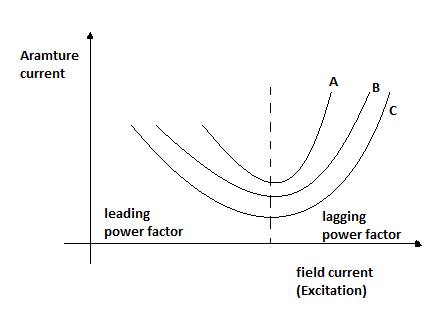# Parallel Operation of Two Alternators - MCQs with answers

## Parallel Operation of Two Alternators - MCQs with answers

Q1. Two identical alternators having impedances Z1 and Z2 are connected in parallel produces E1 and E2 as their induced emf’s. if no load is connected to the alternators then a circulating current will flow in the circuit. This current is given by

a. E1 + E2 / Z1 - Z2
b. E1 - E2 / Z1 + Z2
c. E2 - E1 / Z1 + Z2
d. E1 + E2 / Z1 + Z2

ANSWER: b. E1 - E2 / Z1 + Z2

Q2. If a 3 phase alternator is short circuited at its terminal, then the initial value of short circuit current will be limited by

a. Synchronous reactance
b. Transient reactance
c. Sub transient reactance
d. All of the above

ANSWER: c. Sub transient reactance

Q3. The change in excitation of the two alternators operating in parallel causes

a. Only the kVAR sharing of two alternators without disturbing kW sharing of the two machines
b. Only the kW sharing of two alternators without disturbing kVAR sharing of the two machines
c. Both the kVAR sharing and kW sharing of two alternators
d. None of the above

ANSWER: a. Only the kVAR sharing of two alternators without disturbing kW sharing of the two machines

Q4. In case of hydro generators, the driving torque can be changed by controlling the

a. Gate opening
b. Throttle opening
c. Both (a) and (b)
d. None of the above

ANSWER: a. Gate opening

Q5. The increase in mechanical torque in case of alternator will

a. Increase the armature current and reduce the power factor
b. Increase the armature current and improve the power factor
c. Decrease the armature current and improve the power factor
d. Decrease the armature current and reduce the power factor

ANSWER: b. Increase the armature current and improve the power factor

Q6. When an alternator is connected to infinite bus bar, change in excitation for alternator will

a. Change the terminal voltage and power factor both
b. Affect only terminal voltage and power factor remains unaffected
c. Affect only power factor and terminal voltage remains unaffected
d. Neither terminal voltage will get affected nor power factor

ANSWER: c. Affect only power factor and terminal voltage remains unaffected

Q7. The plot of armature current verses excitation is known as V curves for synchronous generator. Determine the correct combination of load.a. A – full load, B – half load, C – no load
b. A – full load, B – no load, C – half load
c. A – no load, B – half load, C – full load
d. A – half load, B – full load, C – no load